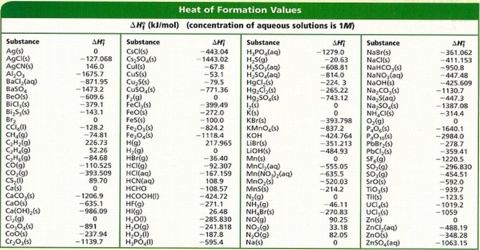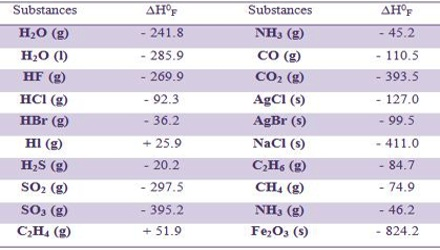# Heat of Formation

Heat of Formation

“The standard heat of formation of a compound is defined as the change in hear content, ∆H when one mole of the substance is formed from its elements in the most stable form in their standard stares”. The heat of formation is usually denoted by ΔHf. It is typically expressed in units of kilojoules per mole (kJ/mol).

In chemistry, the heat of formation is the heat released or absorbed (enthalpy change) during the formation of a pure substance from its elements at constant pressure. It is the amount of heat absorbed or evolved when one mole of a compound is formed from its constituent elements, each substance being in its normal physical state (gas, liquid, or solid). In calculating heats of formation, the enthalpies of the elements in their standard states are arbitrarily taken as zero. For example, in the reaction –

C (graphite) + O2 (g) → CO2 (g); ∆H0 = – 393.5 kJ

it is implied that the heat of formation of carbon dioxide is – 393.5 kJ because 393.5 kJ of heat is evolved when one mole of carbon dioxide gas is formed at 1-atmosphere pressure and 250 C from carbon (graphite) and oxygen under the same conditions. Usually, the conditions at which the compound is formed are taken to be at a temperature of 25 °C (77 °F) and a pressure of 1 atmosphere, in which case the heat of formation can be called the standard heat of formation. As the heat of formation of a compound is the difference between the heat content of the compound and that of its elements and since the heat of its element (graphite and oxygen in the above example) is taken as zero by convention, it follows that the heat of formation of the compound is equal to its heat content. However, the heat of formation of a pure element has a value of 0.

The standard heats of formation of many organic and inorganic compounds have been compiled. A few of them are given in Table.

Table: Heat of formation of some compounds at 250 C in kJ mol-1The heats of formation data are very useful in calculating enthalpy changes of reactions which are difficult to measure directly. The change in enthalpy ∆H of a given reaction may be obtained by subtracting the heats of formation, ∆H0F, of the reactant from those of the products. The first step in this process will be the negative of the sums of the enthalpies of formation of the reactants. Negative since it is the reverse of the formation reaction (compounds to elements). The second step is the sum of the enthalpies of formation of the products.

∆H0reaction = ∑ (∆H0F)products – ∑ (∆H0F)reactants

In using this formula the number of moles of each of the reactants and products in the balanced equation for the reaction has to be considered. The heat of formation is the change in enthalpy when one mole of a compound is formed at 25 degrees Celsius and 1 atm from its elements. It has been measured in labs and recorded so that we can look it up when we need it.